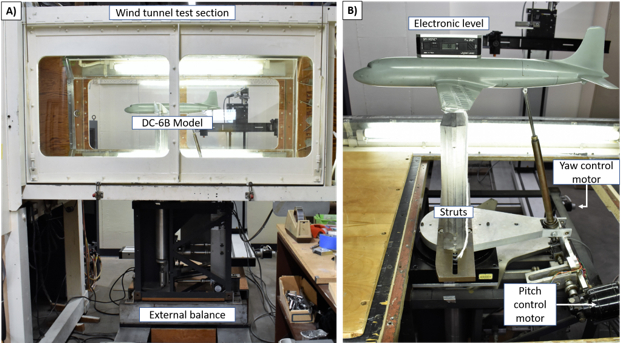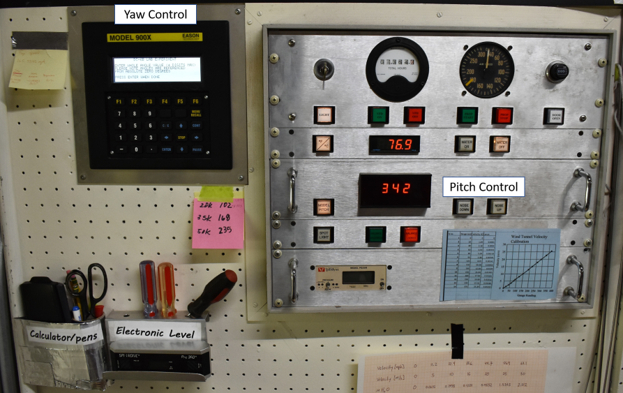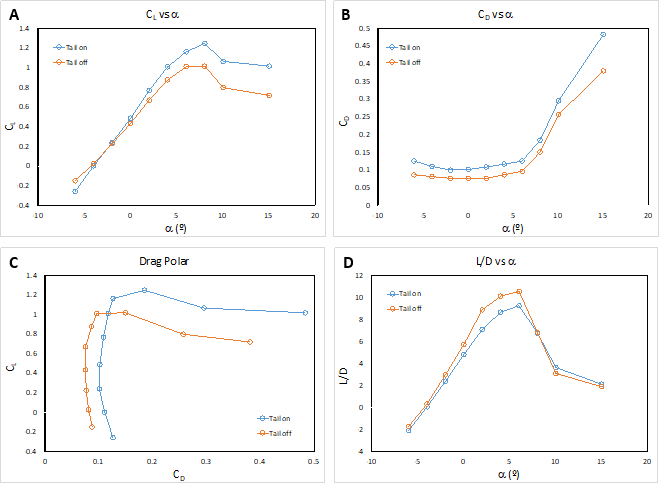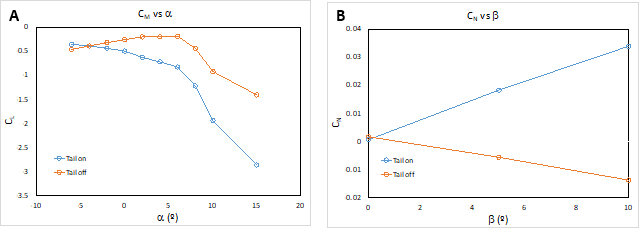Trial ends in

# Aerodynamic Performance of a Model Aircraft: The DC-6B

### Overview

Source: Jose Roberto Moreto and Xiaofeng Liu, Department of Aerospace Engineering, San Diego State University, San Diego, CA

The low-speed wind tunnel is a valuable tool to study aircraft aerodynamic characteristics and evaluate aircraft performance and stability. Using a scale model of a DC-6B aircraft that has a removable tail and a 6-component external aerodynamic force balance, we can measure the lift coefficient (CL), drag coefficient (CD), pitching moment coefficient (CM), and yaw moment coefficient (CN) of the model airplane with and without its tail and evaluate the effect of the tail on aerodynamic efficiency, longitudinal stability and directional stability.

In this demonstration, airplane aerodynamic characteristics and flight performance and stability are analyzed using the aerodynamic force balance measurement method. This method is widely used in aerospace industries and research labs for aircraft and rocket development. Here, a model DC-6B airplane is analyzed at different flow conditions and configurations, and its behavior is analyzed when it is subjected to sudden changes.

### Principles

To evaluate aerodynamic characteristics, it is important to determine how the aerodynamic coefficients change with respect to the airplane attitude, i.e., the angle of attack, yaw angle and roll angle, for a given flight condition. The aerodynamic force balance is a widely used method to directly measure the forces and moments experienced by a model. From the measured forces and moments, as well as the airflow temperature, static pressure and total pressure, the aerodynamic coefficients can be obtained for several angles of attack and yaw angles.

It is possible to obtain the aerodynamic characteristics of a full-scale object by testing a small-scale model, provided that the dynamic similarity condition is met and the appropriate corrections are applied. In the case of an incompressible steady flow, the relevant similarity parameter is the Reynolds number based on a proper reference length.

For a low speed airplane, like the DC-6B, the aerodynamic characteristics can be measured in a small, low-speed wind tunnel since it is possible to match the Reynolds number for the same flight conditions. Under these conditions, one can obtain the dependence of drag and lift on the angle of attack, α. This dependence on alpha can be used to evaluate the airplane performance.

Once the aerodynamic coefficients are measured for several conditions and configurations, for example using two different tail geometries, the stability derivatives (dCM/, dCN/), lift slope (dCL/), maximum lift coefficient, maximum lift-to-drag ratio, and other aerodynamic characteristics can be found. From these aerodynamic coefficients, the effect of modification or design choices on the airplane stability and performance can be determined.

The stability derivatives indicate whether the aircraft is stable or unstable. For example, if the angle of attack of the aircraft suddenly increases due to a gust of wind, the response of the aircraft characterizes its stability. If the angle of attack continues increasing indefinitely, the aircraft is said to be unstable. However, if the angle of attack returns to its initial value, the attitude before the gust, the aircraft is said to be stable. The same is true for the directional stability; if the tendency of the aircraft is to return to its initial yaw angle after a sudden change, the aircraft is said to be directionally stable.

In this demonstration, the aerodynamic force balance for force and moment measurements in a wind tunnel, will be introduced. To remove the contributions of the supporting struts and the model's weight, the balance will be tared to ensure the final results on aerodynamic force and moments are due to the aircraft only. Additionally, this demonstration illustrates the effect of a tail in a conventional airplane design and its importance in longitudinal and lateral aircraft stability.

### Procedure

The DC-6B model set-up on the aerodynamic force balance is displayed below.Figure 1. Mounted DC-6B model. A) DC-6B model inside low-speed wind tunnel test section with an external aerodynamic balance. B) DC-6B Model mounted on the balance by three articulated points. There is also a yaw angle control motor, pitch control motor and an electronic level to calibrate the pitch angle.Figure 2. Low speed wind tunnel control panel. The pitch angle and the yaw angle can be electronically controlled from the panel during the tests with the wind tunnel running.

1. Calibration of set-up

1. Lock the external balance on the wind tunnel control panel.
2. Install the struts on the aerodynamic balance as illustrated in Figure 1. The struts are bolted to the balance.
3. Set the yaw angle to zero by adjusting the knob on the yaw motor, and set the pitch angle to zero using the pitch motor. The pitch angle should be calibrated using an electronic level. The measurements are first made at different angles with only the struts in place, and no model airplane. This enables the effects of the struts from the airplane to be subtracted.
4. Turn on the computer and external balance force acquisition system. It is necessary to turn on the system at least 30 min prior testing.
5. Open the measurement control software.
6. Record the room pressure and temperature. Make sure to correct the barometric pressure using the local temperature and local gravity.
7. Check if the test section and wind tunnel are free from debris and loosen parts, then close the test section doors.
8. Unlock the external balance and set the wind tunnel speed to zero.
9. Turn on the wind tunnel and wind tunnel cooling system.
10. Record the balance forces and moments.
11. Set the dynamic pressure to 7 inches H2O, and record the balance forces and moments.
12. Use the yaw control to set the yaw angle to 5°. Adjust the dynamic pressure to 7 inches H2O if necessary.
13. Record the balance forces and moments. Change yaw angle to 10°. Adjust the dynamic pressure to 7 inches H2O if necessary.
14. Record the balance forces and moments.
15. Turn off the wind tunnel and lock the external balance.
16. Install the DC-6B model with the tail on.
17. Calibrate the angle of attack and pitch indicator. Calibrate the pitch angle prior to the test using an electronic level.
18. Unlock the external balance.
19. Set the angle of attack by pressing the nose up or down on the control panel Figure 2. Angles of attack for testing α = -6°, -4°, -2°, 0°, 2°, 4°, 6°, 8°, 10°.
20. Record the balance forces and moments.
21. Repeat steps 1.19 to 1.20, incrementally increasing the angle of attack until all the test points are complete.
22. Return the angle of attack, α, to zero and set yaw angle. Yaw angles for testing β = 0°, 5°, 10°.
23. Record the balance forces and moments.
24. Repeat steps 1.22 to 1.23 incrementally increasing the yaw angle until all the test points are complete.
25. Lock the external balance and remove the tail from the DC-6B model. Install the tail cone, and repeat steps 1.19 to 1.24.
26. When all data has been collected, turn off the wind tunnel cooling system, lock the external balance, and turn off the wind tunnel.

2. Testing at non-zero wind speeds

1. Check if the test section and wind tunnel are free from debris and loosen parts and then close the test section doors.
2. Set pitch angle to zero.
3. Unlock the external balance.
4. Set the wind tunnel speed dial to zero and turn on the wind tunnel and wind cooling system.
5. Record the balance forces and moments.
6. Set dynamic pressure to 7 inches H2O.
7. Set angle of attack, starting with α = -6°. Angles of attack for testing α = -6°, -4°, -2°, 0°, 2°, 4°, 6°, 8°, 10°.
8. Adjust dynamic pressure to 7 inches H2O if necessary, and record the balance forces and moments.
9. Repeat steps 2.7 - 2.8 incrementally increasing the angle of attack until all the test points are executed.
10. Return the angle of attack to zero and set the yaw angle. The following yaw angles should be tested β = 0°, 5°, 10°.
11. Adjust dynamic pressure to 7 inches H2O if necessary, and record the balance forces and moments.
12. Repeat steps 2.10 - 2.11 incrementally increasing the yaw angle until all test points are executed.
13. Slowly decrease the airspeed to zero, then lock the external balance.
14. Remove the DC-6B model tail cone and install the complete tail.
15. Repeat steps 2.7 to 2.12.
16. When all data has been collected, turn off the wind tunnel cooling system, lock the external balance, and turn off the wind tunnel.

In order to operate an aircraft in three dimensions, we must be able to control its attitude, or orientation, in three dimensions. Thus, we define three principal axes to describe an airplane's position and any changes made to it. The origin of these three axes is located at the aircraft's center of gravity, which is the average location of its mass.

The yaw axis is perpendicular to the aircraft's wings and describes its motion from side to side. The pitch axis is oriented parallel to the wing and perpendicular to the yaw axis. Pitch motion is the up and down motion of the nose. Finally, the roll axis runs the length of the aircraft and describes the vertical movement of the wings.

To evaluate the aerodynamic characteristics of an aircraft as it changes position in these directions, we can measure several different coefficients that describe lift, drag, and moment. The lift and drag coefficients are dimensionless values that enable us to model the complex effects of shape and flow on lift and drag.

The lift and drag coefficients are defined as shown, where L and D are lift and drag, and S is the reference area of the aircraft model. Rho and V are the density and velocity of the free stream. We can simplify rho V squared over two to the dynamic pressure, q.

Similarly, engineers measure the pitching moment coefficient, which is a dimensionless value that describes the torque produced by forces on the aircraft in the direction of the pitch axis, called the pitching moment.

Like the lift and drag coefficients, the pitching moment coefficient is defined as shown, where M is the pitching moment, q is the dynamic pressure, and S and C are the reference area and reference length of the aircraft.

Finally, we can measure the yaw moment coefficient, which describes the torque produced in the direction of the yaw axis. This coefficient is defined as shown, where N is the yaw moment, and B is the wingspan on the aircraft.

Engineers use these coefficients to study aircraft performance and stability. The stability derivatives, taken with respect to the pitch or yaw angles, indicate whether the aircraft is stable or unstable.

For example, if the angle of attack, alpha, is suddenly increased by a wind gust, the aircraft's response determines its stability.If the angle of attack keeps increasing indefinitely, the aircraft is unstable. This is shown by a positive stability derivative, showing that the pitching moment coefficient continues to increase with alpha.

The same is true for directional instability with respect to yaw angle beta, which gives a negative stability coefficient. If the angle of attack or yaw angle return to their initial values, then the aircraft is said to be stable. This is reflected in the stability derivatives, which are opposite to the unstable conditions.

In this experiment, we will examine a model aircraft as it is exposed to airflow at different pitch and yaw angles and determine its stability and performance with and without its tail.

In this experiment, we will examine a model aircraft as it is exposed to airflow at different pitch and yaw angles and determine its stability and performance with and without its tail.

For this experiment, you'll need to use an aerodynamic wind tunnel with a force balance that controls the angle of attack, also called the pitch angle, and the yaw angle externally during the experiment. You'll also need a DC-6B aircraft model that attaches to the force balance using struts.

To begin, lock the external balance and install the struts on the balance to analyze the effects of the struts alone, so they can be subtracted out of the airplane measurements. Set the yaw angle to 0 by adjusting the yaw motor knob.

Now turn on the computer and turn on the data acquisition system for the external force balance. Allow the system to warm up for 30 min prior to testing.

Once the system has warmed up, open the data acquisition software. Read the room pressure and temperature and record these values in your notebook. Correct the barometric pressure, using the barometer spreadsheet that accompanies the mercury barometer.

Now make sure that the test section and wind tunnel are free of debris and loose parts. Then close the test section doors. Unlock the external balance. Then set the wind tunnel speed dial to 0. Turn on the wind tunnel and the wind tunnel cooling system. Record the balance forces and moments with the wind speed at 0.

Now adjust the yaw angle to 5° using the yaw control. Then record the balance forces and moments again at 0 wind speed. Repeat these measurements again at a yaw angle of 10° and zero wind speed. Now set the yaw angle back to 0 and then set the dynamic pressure to 7 inches of water. Then record the balance forces and moments again.

Now, set the yaw angle to 5°, adjust the dynamic pressure back to 7 inches of water, if necessary, and then record the balance forces and moments. Repeat the same measurements at a yaw angle of 10°, resetting the dynamic pressure back to 7 inches of water, if necessary.After the measurements have been recorded, return the yaw angle to zero, and turn off the wind tunnel.

To begin calibration of the model DC-6B airplane, first lock the external balance and open the test section. Then install the DC-6B model with the tail on. Calibrate the pitch angle using an electronic level and make adjustments to zero if needed.

After closing the test section doors, unlock the external balance, press the nose down button to set the pitch angle to -6°. Now record the balance forces and moments with the wind tunnel off to acquire the correction needed to account for the model's weight.

Change the pitch angle to -4° and repeat the measurement of the force and moments as before. Conduct the test for angles of attack up to 10° with 2° increments. Then return the pitch angle to zero. Now conduct the same test for the yaw angles 0,5,and 10°. When all of the angles have been tested, lock the external balance, open the test section, and remove the DC-6B model tail.

Then install the tail cone, so that we can measure the model weight contribution with the wind tunnel off. Now close the test section, set the yaw angle to zero, and record the force and moment measurements for all of the pitch angles from -6 to 10°, as before.

Once those measurements are complete, repeat the test again at a pitch angle of0 for the three yaw angles. When complete, lock the external balance.

Now we'll run the experiment with a non-zero wind speed. To begin, check the test section for debris and loose parts. Then, close the test section doors.

Next, set the pitch angle to zero and unlock the external balance. Set the wind tunnel speed dial to zero, then turn on the wind tunnel. Record the balance forces and moments before turning on the airflow. Now turn on the airflow with the dynamic pressure equal to 7 inches of water. Then set the pitch angle to -6°, and adjust the dynamic pressure back to 7 inches of water, if needed, before recording the balance forces and moments for this setting.

Repeat the measurement for each of the pitch angles tested in the calibration steps. Then return the pitch and yaw angles to zero. Adjust the dynamic pressure again if needed, and then record the balance forces and moments. Like before, repeat the measurements for the yaw angles tested during the calibration.

Once all of the measurements have been taken, slowly decrease the air speed to zero. Now lock the external balance and open the test section. Remove the DC-6B tail cone and install the complete tail. Then close the test section and repeat the measurements for all of the pitch angles and yaw angles tested previously with a wind tunnel dynamic pressure of 7 inches of water.

In this experiment, we obtained performance and stability characteristics of a DC-6B aircraft model in two configurations, with the conventional airplane tail and with the tail removed.

For each configuration, adjust the measured forces to remove the weight of the strut by subtracting the forces with the model off and wind off from the forces with the model off and the wind on.

Then remove the effect of the weight of the model by subtracting the forces with model on and wind off from the forces with the model on and wind on. Then remove the aerodynamic effect of the struts by subtracting the weight adjusted forces of the struts from the weight adjusted forces of the model.

Using these adjusted forces, we can calculate the lift coefficient and drag coefficient using these equations. Here, L is the lift and D is the drag, which were measured in the experiment. S is the model reference area and q is the dynamic pressure.

Now if we plot the lift and drag coefficients against the pitch angle, we can see that the tail on the aircraft increases the maximum lift, but the tail also increases the drag. Next, let's look at the pitching moment coefficient.The pitching moment, M, was measured in our experiments.

Then, we'll plot the pitch moment coefficient against the pitch angle. Remember that if the pitch moment increases with increasing angle of attack, the aircraft is unstable, as it is unable to return to level heading. But if the pitch moment decreases with increasing angle of attack, the pitch moment acts to prevent the pitch angle from increasing or decreasing indefinitely; thus, ensuring more stability in the aircraft.

For the tail off configuration, the pitch coefficient increases with the increase of the pitch angle, showing that the aircraft is unstable in this configuration. On the other hand, the tail on configuration exhibits the opposite behavior, where the pitch coefficient decreases as the pitch angle increases, showing that the tail adds stability to the aircraft.

Similarly, we will calculate the yaw moment coefficient. The yaw moment, N, was measured in our experiments. Here we show a plot of the yaw moment coefficient versus the yaw angle.

For directional stability, a positive side slip angle beta means that the aircraft nose is pointing to the left of the direction of motion, and to the right if beta is negative. The yaw moment coefficient is positive to the right and negative to the left.

However, if the yaw moment decreases as beta increases, as it does for the tail off configuration, the airplane does not tend to return to the zero beta position and is unstable. Therefore, we can conclude that the airplane tail is necessary to achieve stability, even though it results in some performance reduction.

In summary, we learned how the aerodynamic characteristics of an aircraft are described by its lift, drag, and moment coefficients. We then measured the aerodynamic forces experienced by model DC-6B airplane in a wind tunnel to analyze its flight performance and stability.

### Results

In this demonstration, the performance and stability characteristics of a DC-6B model in two configurations were measured. In one configuration, a conventional airplane tail was attached to the model (tail-on), and in the second configuration, the tail was removed and replaced with a cone (tail-off). For each configuration, the variation of lift coefficient and drag coefficient with angle of attack was determined (Figure 3). The variation in pitch moment coefficient and yaw moment coefficient with respect to angle of attack and beta was also investigated (Figure 4).

The results show the aerodynamic effects of the tail. In Figure 3, although the tail increases the maximum lift and the drag, overall the tail decreases the aerodynamic performance. When the tail is off, the model is longitudinally and directionally unstable (Figure 4). Therefore, the airplane tail is necessary to achieve stability, even though it could result in reduced aircraft performance.Figure 3. Performance evaluation curves for tail-on and tail-off configurations. A) Lift coefficient vs α; B) Drag coefficient vs α; C) Drag polar; and D) L/D vs α. Please click here to view a larger version of this figure.Figure 4. Performance evaluation curves for tail on and tail off configurations. A) Pitch moment coefficient vs α; B) Yaw moment coefficient vs β. Please click here to view a larger version of this figure.

### Applications and Summary

Testing a small-scale model using an aerodynamic balance in a wind tunnel enables the determination of major aerodynamic characteristics of an aircraft. A 6-component balance measures three force components, lift, drag, and lateral forces, and three moment components, pitch, yaw, and roll moments.

When the dynamic similarity between the full-scale object and the model is achieved, for example the Reynolds number is the same for the case of incompressible steady flow, then the aerodynamic coefficients obtained using the small-scale model are applicable to the full-scale object and aerodynamic characteristics, such as performance and static stability, can be determined.

Force and moments measurements by an external balance in a wind tunnel have several applications. This method is widely used in the aerospace industry; however, it has been applied successfully in research and development in many areas, for example in naval engineering, automotive industries, and civil engineering.

There are several applications in naval engineering. For example, sail boats and race boats are significantly affected by aerodynamic forces, and their effect on the vessel needs to be considered to optimize performance. For low-speed ship design, aerodynamic forces should be considered to reduce fuel consumption and improve overall performance.

Another industry that benefits from wind tunnel testing is the automotive industry. Wind tunnel testing is used to determine the drag forces, lateral forces and moments experienced by a car. This is now a standard practice for the development of new cars since this technique leads to more competitive and efficient designs.

Wind tunnel testing for force measurements is not restricted to performance optimization. In the modern civil engineering industry, wind tunnel tests are used to increase safety. There are tall and slender skyscrapers which are subject to strong wind gusts. These wind gusts generate high loads that need to be accounted for in building design to avoid building collapse. This also applies to bridges, which must be tested in wind tunnels to ensure safety.

Materials List:

 Name Company Catalog Number Comments Equipment Low-speed wind tunnel SDSU Closed return type with speeds in the range 0-180 mph Test section size 45W-32H-67L inches DC-6B full model SDSU Reference area = 93.81 in2 Mean Chord Length = 3.466 in Span = 27.066 in Aspect Ratio = 7.809 Moment Reference Z-Distance (in) = 0* Moment Reference X-Distance (in) = 0* External Aerodynamic Force Balance SDSU 6-component, load-cell, strain gauge type balance system has the following load limits. Lift = 150 lb; Drag = 50 lb; Side Force 100 lb; Pitch 1000 lb-in; Roll 1000 lb-in; Yaw 1000 lb-in. Digital Service Module Scanivalve DSM4000 Barometer Manometer Meriam Instrument Co. 34FB8 Water manometer with 10” range. ThermometerX